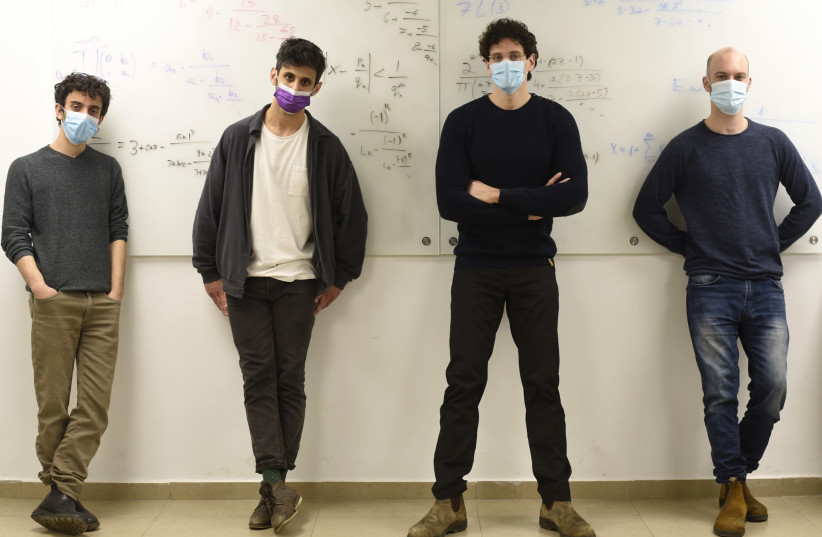(function (a, d, o, r, i, c, u, p, w, m) { m = d.getElementsByTagName(o), a[c] = a[c] || {}, a[c].trigger = a[c].trigger || function () { (a[c].trigger.arg = a[c].trigger.arg || []).push(arguments)}, a[c].on = a[c].on || function () {(a[c].on.arg = a[c].on.arg || []).push(arguments)}, a[c].off = a[c].off || function () {(a[c].off.arg = a[c].off.arg || []).push(arguments) }, w = d.createElement(o), w.id = i, w.src = r, w.async = 1, w.setAttribute(p, u), m.parentNode.insertBefore(w, m), w = null} )(window, document, "script", "https://95662602.adoric-om.com/adoric.js", "Adoric_Script", "adoric","9cc40a7455aa779b8031bd738f77ccf1", "data-key");
Technion researchers develop mathematical 'conjecture generator' - The Jerusalem Post
!function(n){if(!window.cnxps){window.cnxps={},window.cnxps.cmd=[];var t=n.createElement('iframe');t.display='none',t.onload=function(){var n=t.contentWindow.document,c=n.createElement('script');c.src='//cd.connatix.com/connatix.playspace.js',c.setAttribute('async','1'),c.setAttribute('type','text/javascript'),n.body.appendChild(c)},n.head.appendChild(t)}}(document);

# Technion researchers develop mathematical 'conjecture generator'

## The machine was named after the Indian mathematician Ramanujan, who came from a poor family but nevertheless had a major impact on the formulation of unproven mathematical formulas.The Technion research team responsible for developing the conjecture generator
(photo credit: TECHNION SPOKESPERSON'S OFFICE)
Researchers from the Technion-Israel Institute of Technology have developed a "conjecture generator" that creates mathematical conjectures using a combination of artificial intelligence and computer automation, according to the university on Thursday.
Called the Ramanujan Machine, a study about the machines' development was published in the academic journal Nature, and was carried out by undergraduates from a variety of faculties under the guidance of Prof. Ido Kaminer from the Andrew and Erna Viterbi Faculty of Electrical Engineering at the Technion.
The project focused on mathematical constants, which are fixed values that originate from different mathematical calculations and structures in different fields. The importance of the mathematical constants apply to fields with a strong use of math, such as biology, physics and ecology.
One of the most well-known constants is pi, the circumference of a circle, which has been studied since antiquity. The development of a conjecture machine seeks to find mathematical conclusions to problems that have yet to be solved, which when proven, can become a theorem.
The machine was named after the Indian mathematician Ramanujan, who came from a poor family but nevertheless had a major impact on the formulation of unproven mathematical formulas.
“Our results are impressive because the computer doesn’t care if proving the formula is easy or difficult, and doesn’t base the new results on any prior mathematical knowledge, but only on the numbers in mathematical constants," said Kaminer.
"To a large degree, our algorithms work in the same way as Ramanujan himself, who presented results without proof. It’s important to point out that the algorithm itself is incapable of proving the conjectures it found – at this point, the task is left to be resolved by human mathematicians,” he added.
cnxps.cmd.push(function () { cnxps({ playerId: '36af7c51-0caf-4741-9824-2c941fc6c17b' }).render('4c4d856e0e6f4e3d808bbc1715e132f6'); });
if(window.location.pathname.indexOf("656089") != -1){console.log("hedva connatix");document.getElementsByClassName("divConnatix").style.display ="none";}
The new machine has already started delivering new conjectures, leading to new formulas for prominent mathematical constants such as pi, Euler’s number (e), Apéry’s constant (which is related to the Riemann zeta function), and the Catalan constant. To publicize the machine’s capabilities, the researchers launched a website, RamanujanMachine.com, to allow the public to be more involved in advancing mathematical research.

Subscribe for our daily newsletterBy subscribing I accept the terms of use